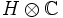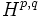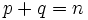Pure Hodge structure

(Redirected from Hodge structure)
Let$H$ be an Abelian group. A pure Hodge structure of weight$n$ on$H$ is a decomposition of$H \otimes \mathbb{C}$ as a direct sum of components$H^{p,q}$ where$p,q$ are nonnegative and$p + q = n$, such that$\overline{H^{p,q}} = H^{q,p}$.